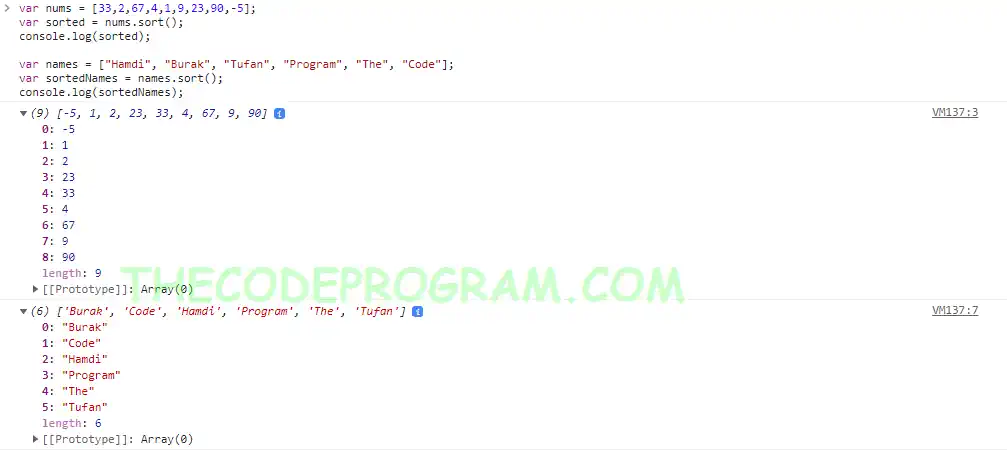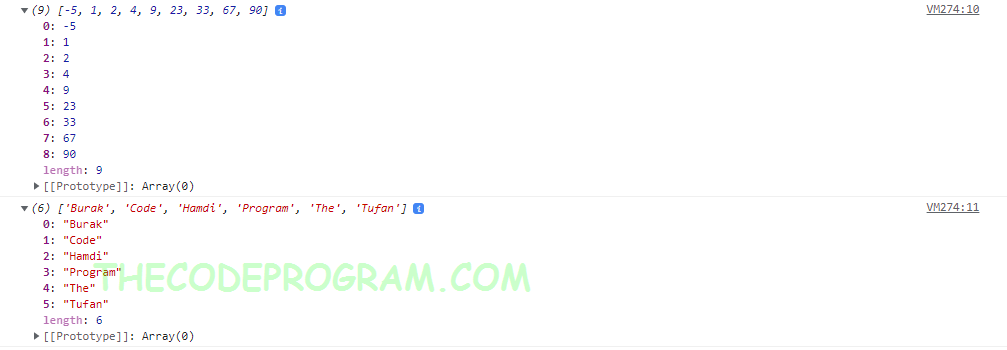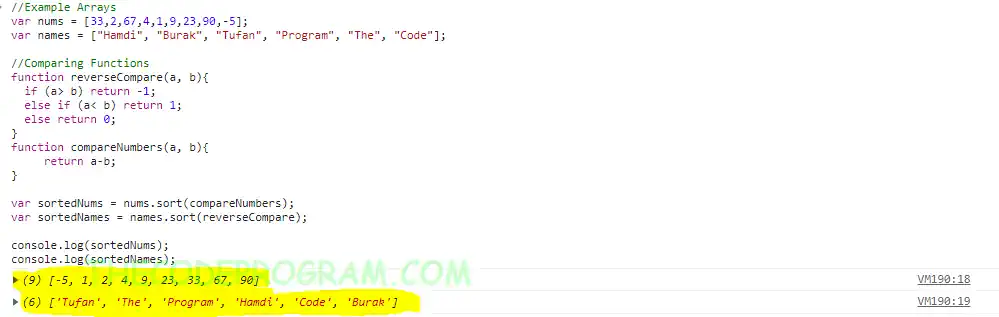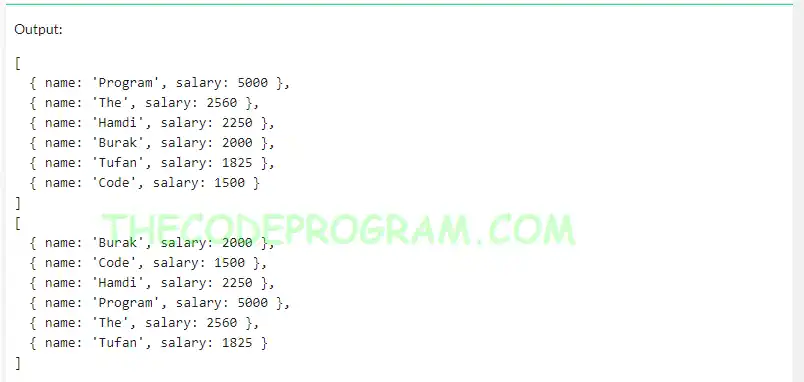## Explanation of Array sort method in JavaScript

Hello everyone, in this article we are going to talk about object sorting in JavaScript language. We use sort() method to an array with specified comparing function.

Let's begin.

#### Array sort() method

sort() method returns the given array after sorting operation. It sort the array in ascending order in default condition. If we define a custom compare function, array will be sorted according to given sorting function.

There are three ways to sort an array with sort() method:
• sort() : We can directly sort an array in ascending order with no sorting function.
• sort(compareFunction(a, b)) : We can use a comparing function for sorting the elements according to requirements. We decalre the function in a location and we can call in here. Also we can declare the compare function inline.
• sort((a, b) => { /*comparing logic*/ } ) : We can also use arrow declaration. We need to define the comparing logic for sorting algorithm.

All of above comparing methods returns the sorted array. According to specified comparing logic.

Now let's sort some arrays with sort method directly
``````
var nums = [33,2,67,4,1,9,23,90,-5];
var sorted = nums.sort();
console.log(sorted);

var names = ["Hamdi", "Burak", "Tufan", "Program", "The", "Code"];
var sortedNames = names.sort();
console.log(sortedNames);
``````
Below you can see the example output:Now let's sort an array with arrow function in our sort function:
``````
var nums = [33,2,67,4,1,9,23,90,-5];
var names = ["Hamdi", "Burak", "Tufan", "Program", "The", "Code"];
var sortedNums = nums.sort((a, b) =>  a>=b ? 1 : -1);
var sortedNames = names.sort((a, b) => {
if (a> b) return 1;
else if (a< b) return -1;
else return 0;
});

console.log(sortedNums);
console.log(sortedNames);
``````
Below iage you can see the example output:Now let's make a sorting example with function:
``````
//Example Arrays
var nums = [33,2,67,4,1,9,23,90,-5];
var names = ["Hamdi", "Burak", "Tufan", "Program", "The", "Code"];

//Comparing Functions
function reverseCompare(a, b){
if (a> b) return -1;
else if (a< b) return 1;
else return 0;
}
function compareNumbers(a, b){
return a-b;
}

var sortedNums = nums.sort(compareNumbers);
var sortedNames = names.sort(reverseCompare);

console.log(sortedNums);
console.log(sortedNames);
``````
Below image you can see the example output:We can also sort JSON objects with sort method in JavaScript. We need to declare a sorting function to compare the field that objects will be sorted. We can declare both arrow and normal function to sort objects.

Now let's make an example about object sorting.
``````
var employees = [
{ name: 'Burak', salary: 2000 },
{ name: 'Hamdi', salary: 2250 },
{ name: 'Tufan', salary: 1825 },
{ name: 'The', salary: 2560 },
{ name: 'Code', salary: 1500 },
{ name: 'Program', salary: 5000 }
];
var salarySorted= employees.sort((a, b) =>  a.salary>=b.salary ? -1 : 1);
console.log(salarySorted);

var nameSorted= employees.sort((a, b) => {
if (a.name> b.name) return 1;
else if (a.name< b.name) return -1;
else return 0;
});
console.log(nameSorted);
``````
Below image you can see the example outputHave a good data sorting

Burak Hamdi TUFAN

24/05/2020

27/02/2021

21/02/2022